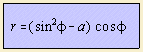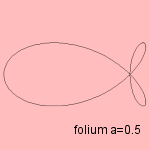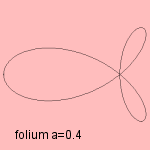# folium

## quartic1)

The folium 2 has three forms:

•a >= 1: single folium or simple folium

Some authors confine the simple folium to a = 1.
In this case the equation of the curve can be rewritten as r=cos3φ.
The curve is the inverse of Tschirnhausen's cubic.
• a = 0: regular bifolium (or regular double folium)
The curve is sometimes called the bifolium, but I see the curve as a special case of this bifolium.
Alternative names for the curve are: right bifolium, right double folium, bifoliate, and rabbit-ear3.The Cartesian equation of the curve can be written as y = ± √x ± √x(1-x).

The curve can be constructed with a given circle C through O as follows: draw for each point Q on C points P, so that PQ = OQ. Then the collection of the points P forms the regular bifolium.
Another construction is as the mediane curve of a parabola and an ellipse.

The curve can be generalized to the generalized regular bifolium.
• 0 < a < 1: trifolium
For a=1/2 the curve is called the trefoil4, torpedo curve5 or siluroid (see the website of Dario de Judicibus).
Its equation can be written as r = cos2φ cosφ or as r = sin4φ sinφ.A Cartesian form of its equation is (x2+y2)2 = x(x2-y2).

For a=1/4 we see the regular trifolium, which is in fact a rosette.

Each of the three folia is a (different) pedal of the deltoid.

It was Johann Kepler (1609) who was the first to describe the curve.
Therefore the curve is also known as Kepler's folium.notes

1) In Cartesian coordinates: (x2 + y2)2 + a x3 = (1-a) x y

2) Folium (Lat.) = leaf.

3) In French: oreilles de lapin.

5) In French: torpille.